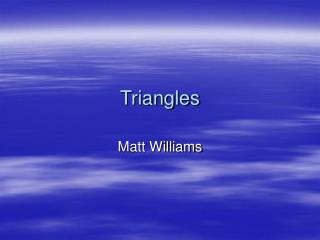DownloadDownload PresentationTriangles

# Triangles

Télécharger la présentation## Triangles

- - - - - - - - - - - - - - - - - - - - - - - - - - - E N D - - - - - - - - - - - - - - - - - - - - - - - - - - -
##### Presentation Transcript

1. Triangles Matt Williams

2. The Definition of a Triangle • A figure formed by connecting three non-collinear points. • A triangle has three sides and three angles.The sum of all the angles equals 180 degrees. • Triangles are characterized by the length of their sides or the measure of their angles. There are six types of triangles, three in each of these groups. • The triangles characterized by the length of their sides are Isosceles, Equilateral, and Scalene triangles. • The triangles characterized by the measure of their angles are Acute, Right, and Obtuse triangles.

3. Triangles – Length of Sides • An equilateral triangle is one where the length of all the sides are equal. • An isosceles triangle features two sides of equal length. • A scalene triangle is made of sides that are not equal. All the sides are different lengths.

4. Triangles – Measure of Angles • Remember that the sum of the angles in any triangle equals 180 degrees. • A right triangle contains an angle that equals 90 degrees. This angle is shown with a square or box in that angle. • In an acute triangle all of the angles measure less than 90 degrees. • An obtuse triangle has one angle with a measure of larger than 90 degrees. • An equiangular triangle has all angles with equal measures (must be 60 degrees), and therefore also must have all the lengths of it’s sides equal (meaning it’s an equilateral triangle as well.

5. Pythagorean Theorem • The Pythagorean Theorem is used on RIGHT triangles to find the length of an unknown side if you know the lengths of the other two sides. • The side opposite of the right angle is called the Hypotenuse. • The theorem states that a²+b²=c² where “a” and “b” are the sides of the right triangle that contain the right angle and “c” is the hypotenuse.

6. Activities Worksheet - Identifying Triangles Worksheet Answers Pythagorean Theorem All pictures of triangles from: Go Math

7. Resources • Go Math - http://www.gomath.com/htdocs/lesson/triangle_lesson1.htm • Planet Math - http://planetmath.org/encyclopedia/Triangle.html • Fact Monster - http://www.factmonster.com/ipka/A0876325.html • Math - http://www.math.com/school/subject3/lessons/S3U2L2GL.html